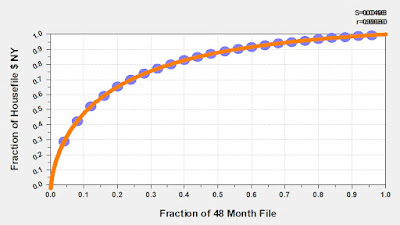## July 19, 2022

### -188 + 192*x

Yeah, yesterday I got frustrated about sample sizes. I'm so tired of people who do not understand math using math to make a point that is vendor-friendly so that vendors make money. It's a form of lying, it is misleading, and it is corrupt.

So let me take you all the way back to Lands' End, in 1991. Yeah, 31 years ago. We were executing tests with 5,000 customers in a cell, and the test results were ALL OVER THE MAP. Not consistent.

I was asked to determine what an appropriate test sample size should be.

I measured the variance of test results at different demand-per-customer levels. There was a clear, linear relationship.

• -188 + 192*(demand-per-customer).
Now I had what I needed to determine if what we were observing might be statistically significant.

Let's say I expected a customer to spend \$5 if catalog-version-one was mailed. I could estimate the variability expected at different sample sizes.
• q = SQRT(2*(-188 + 192*(demand per customer)/(sample size))).
For a result to be statistically significant, the result/difference would have to be greater than 2*q.

Let's assume that demand per customer = \$7.00.
• Sample Size =   5,000:  Q = 0.68.  2*Q = \$1.36.
• Sample Size = 10,000:  Q = 0.48.  2*Q = \$0.96.
• Sample Size = 25,000:  Q = 0.30.  2*Q = \$0.60.
• Sample Size = 50,000:  Q = 0.22.  2*Q = \$0.44.
• Sample Size = 75,000:  Q = 0.18.  2*Q = \$0.36.
If you expected the test group to spend \$0.25 more than the control group (+3.5%), you would need more than 75,000 customers to detect that level of change.

If you expected the test group to spend \$0.70 more than the control group (+10%), you would need about 25,000 customers to detect that level of change.

This is the problem with people executing tests with 8,600 customers. Using the equation above, 2*Q = \$1.04. In other words, unless the test group is at \$8.04 and the control group is at \$7.00 (+15%), you don't know if what you are seeing is due to a change in marketing strategy or is due to complete unfettered random noise.

Again, at every company, this equation is different.

And if you don't know the equation, you don't know if your results make sense or not.

So when I get upset about test results that are published by a reputable university that should know better, this is why I get upset.

P.S.:  If there is a credible statistician reading this, that person should have already sent me an email suggesting that I violated "heteroskedasticity" ... that what I was illustrating was a situation with non-constant variance. Yes! That person would be correct. And that means that my analysis is also flawed ... not that I have a choice because otherwise I could never analyze test results at all, but the point is that the results I illustrate above are likely biased as well.